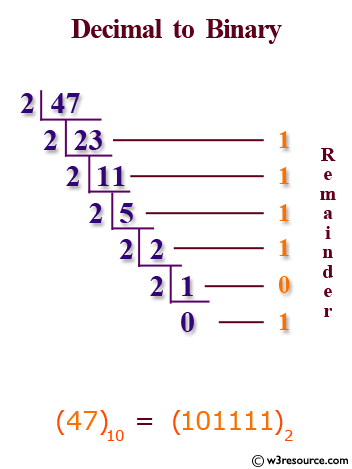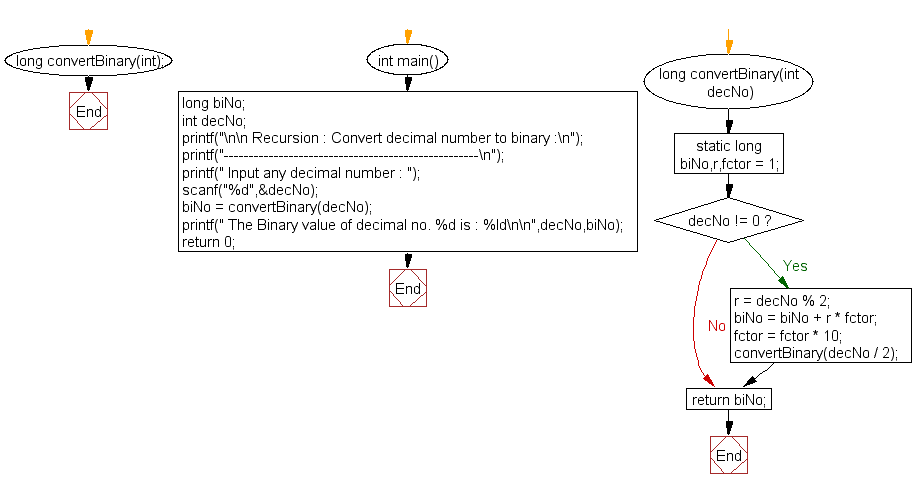﻿ C Program: Convert a decimal number to binary - w3resource

# C Exercises: Convert a decimal number to binary

## C Recursion: Exercise-11 with Solution

Write a program in C to convert a decimal number to binary using recursion.

Pictorial Presentation:Sample Solution:

C Code:

``````#include<stdio.h>

long convertBinary(int);

int main()
{
long biNo;
int decNo;

printf("\n\n Recursion : Convert decimal number to binary :\n");
printf("---------------------------------------------------\n");

printf(" Input any decimal number : ");
scanf("%d",&decNo);

biNo = convertBinary(decNo);//call the function convertBinary
printf(" The Binary value of decimal no. %d is : %ld\n\n",decNo,biNo);
return 0;
}
long convertBinary(int decNo)
{
static long biNo,r,fctor = 1;

if(decNo != 0)
{
r = decNo % 2;
biNo = biNo + r * fctor;
fctor = fctor * 10;
convertBinary(decNo / 2);//calling the function convertBinary itself recursively
}
return biNo;
}
```
```

Sample Output:

``` Recursion : Convert decimal number to binary :
---------------------------------------------------
Input any decimal number : 66
The Binary value of decimal no. 66 is : 1000010
```

Explanation:

```long convertBinary(int decNo)
{
static long biNo,r,fctor = 1;

if(decNo != 0)
{
r = decNo % 2;
biNo = biNo + r * fctor;
fctor = fctor * 10;
convertBinary(decNo / 2);//calling the function convertBinary itself recursively
}
return biNo;
}
```

This function ‘convertBinary()’ takes an integer ‘decNo’ as input and converts it into its binary representation. It does this by recursively dividing the decimal number by 2 and keeping track of the remainders at each step.

The function first initializes a static variable ‘biNo’ to store the binary representation of the decimal number. It also initializes another static variable fctor to keep track of the position of each binary digit.

The function then checks if the input decNo is not equal to zero. If so, it proceeds to compute the remainder r when decNo is divided by 2. It then updates the biNo variable by adding r times the value of fctor. It also updates fctor by multiplying it by 10 to move to the next position of the binary digit.

The function then recursively calls itself with the updated value of decNo divided by 2.

The function continues this recursive process until decNo becomes zero. At this point, it returns the computed binary representation stored in biNo.

Time complexity and space complexity:

The time complexity of the function is O(log n), where n is the input decimal number, since the function recursively divides the input number by 2 until it becomes zero.

The space complexity is also O(log n), as each recursive call adds a new activation record to the call stack.

Flowchart:C Programming Code Editor:

Have another way to solve this solution? Contribute your code (and comments) through Disqus.

﻿

## C Programming: Tips of the Day

__FILE__ macro shows full path

```#include <string.h>

#define __FILENAME__ (strrchr(__FILE__, '/') ? strrchr(__FILE__, '/') + 1 : __FILE__)
For Windows use '\\' instead of '/'.
```

Ref : https://bit.ly/3iEWRoT

We are closing our Disqus commenting system for some maintenanace issues. You may write to us at reach[at]yahoo[dot]com or visit us at Facebook

Test your Programming skills with w3resource's quiz.

﻿

## C Programming: Tips of the Day

__FILE__ macro shows full path

```#include <string.h>

#define __FILENAME__ (strrchr(__FILE__, '/') ? strrchr(__FILE__, '/') + 1 : __FILE__)
For Windows use '\\' instead of '/'.
```

Ref : https://bit.ly/3iEWRoT

We are closing our Disqus commenting system for some maintenanace issues. You may write to us at reach[at]yahoo[dot]com or visit us at Facebook